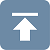[Introduction]  [Signal arithmetic]  [Signals and noise]   [Smoothing]   [Differentiation]  [Peak Sharpening]  [Harmonic analysis]   [Fourier convolution]  [Fourier deconvolution]  [Fourier filter]  [Wavelets]   [Peak area measurement]  [Linear Least Squares]  [Multicomponent Spectroscopy]  [Iterative Curve Fitting]  [Hyperlinear quantitative absorption spectrophotometry] [Appendix and Case Studies]  [Peak Finding and Measurement]  [iPeak]   [iSignal]  [Peak Fitters]   [iFilter]  [iPower]  [List of downloadable software]  [Interactive tools]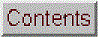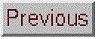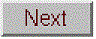## Fourier filter

[Simple Example]   [Command line function]   [Segmented Fourier filter]   [Interactive Fourier Filter]   [Application of a narrow bandpass filter]

The Fourier filter is a type of filtering function that is based on manipulation of specific frequency components of a signal. It works by taking the Fourier transform of the signal, then attenuating or amplifying specific frequencies, and finally inverse transforming the result. In many science measurements, such as spectroscopy and chromatography, the signals are relatively smooth shapes that can be represented by a surprisingly small number of Fourier components. For example, the animation below (script) shows in the top panel a signal of three smooth peaks, with peak heights of 1, 2, and 3, where the x-axis is time in seconds. The middle panel shown the first 51 frequencies of its Fourier spectrum (zero through 50). In this case the x-axis is both the frequency in Hz and the Fourier component number (because the duration of the signal is exactly 1 second). The amplitude of the Fourier components is strongest at low frequencies and drops to near zero at 25 Hz.

z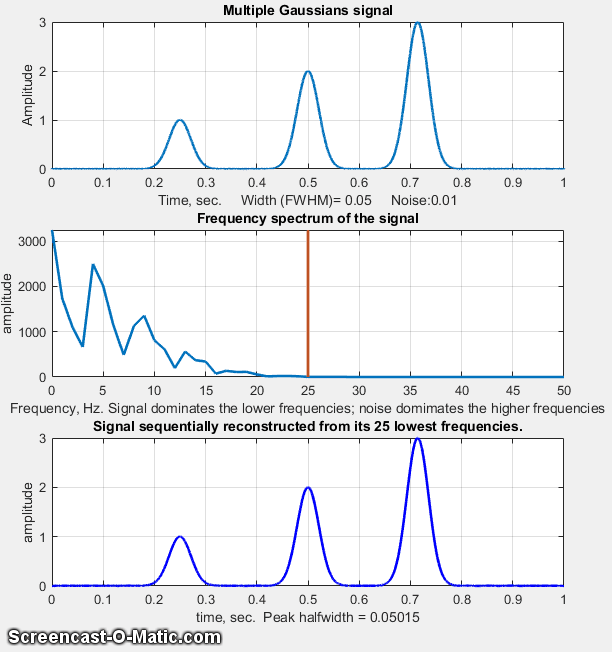The bottom panel shows in this figure the signal re-constructed by inverse transforming the first "n" Fourier components. The animation (visible in a web browser) shows the result of using the frequencies between 1 through 25 progressively. The reconstructed signal starts as a single sine wave and becomes progressively more complex as more frequencies are added, until it is visually indistinguishable from the original signal when 25 frequencies are included. But notice what the reconstructed signal looks like when it gets to 16 frequencies; the amplitude has already dropped very low and there is relatively little amplitude in the remaining frequencies. The three peaks are rendered well but the baseline has a distinct ripple. That is caused by the abrupt cut-off of the frequencies beyond that point, which can be avoided by using a filter with an adjustable filter shape that allows the cut-off rate to be controlled. This is illustrated in the next figure.

The figure below shows the same three-peak signal, but with the addition of random white noise with a standard deviation equal to the the peak height of the smallest peak (line 14 of the same script). White noise has equal amplitude at all frequencies, whereas most of the peak signal is concentrated in the first 25 frequencies, so we can expect that most but not all of the noise can be eliminated by employing a low-pass filter with a cut-off frequency around 25 Hz. The result (bottom panel) shows the three peaks with their position and peak heights approximately intact. Obviously the noise below 25 Hz remains, which is why the signal is imperfect.

The optimization of the Fourier filter for the signal-to-noise (SNR) ratio of peak signals faces the same compromise as conventional smoothing functions; namely, the optimum SNR is achieved when the peak height is less than the noiseless maximum. For example, the script GaussianSNRFrequencyReconstruction.m shows that optimum SNR is reached when the peak height is about half the true value, but the peak area is the same.

A more dramatic example is shown the figure below. In this case, the signal (top left) seems to be only random high-frequency noise, and its Fourier spectrum (top right) shows that high-frequency components dominate the spectrum over most of its frequency range (script). The Fourier spectrum is expanded in the X and Y directions (bottom left) to show more clearly the low-frequency region. There, the series of relatively smooth bumps, with peaks at the 1st, 20th, and 40th frequencies, are most likely the actual signal. Working on the hypothesis that the components above the 40th harmonic are increasingly dominated by noise, the Fourier filter function (FouFilter.m) can be used to gradually reduce the higher harmonics and to reconstruct the signal from the modified Fourier transform (red line). The result (bottom right) shows the signal actually contains two partly overlapping Lorentzian peaks that were totally obscured by noise in the original signal.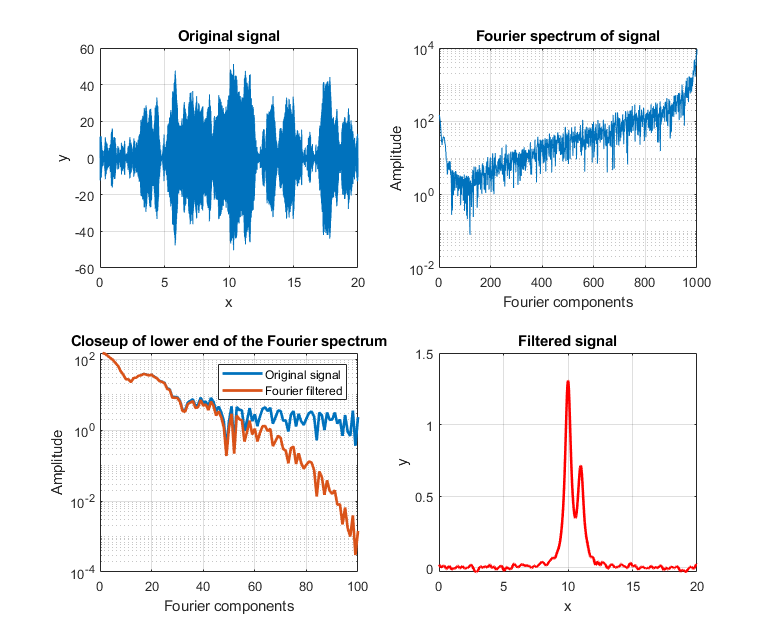Matlab. The simplest possible code for a Fourier filer can be most simply illustrated by a low-pass sharp cut-off filter. Care must be taken to use both the sine and cosine (or equivalently the frequency and phase or the real and imaginary) components of the Fourier transform. The operation must account for the mirror-image structure of the Matlab's Fourier transform: the lowest frequencies are at the extremes of the fft and the highest frequencies are in the center portion. So, to pass the lowest n frequencies, you must pass the first n points and the last n points and zero out the others.

ffty=fft(y); % ffty is the fft of y
lfft=length(ffty); % Length of the FFT
ffty(n:lfft-n)=0; % All frequencies between n and lfft-n in the fft are set to zero.
fy=real(ifft(ffty)); % Real part of the inverse fft

Here, n is the number of frequencies that are passed; all others are simply eliminated. The function form of this operation is flp.m. This is the minimal essence of a Fourier filter. The script flptest.m demonstrates its use (figure on the right). The is not really a practical filter, however, because its abrupt cutoff usually results in ringing on the baseline, as shown above.General-purpose Fourier filter function. To make the Fourier filter more generally useful, we must add code to include not only low-bass, but also high-pass, band pass, and band reject filter modes, plus a provision for more gentle and variable cut-off rates. This, and more, is done in the in the following section. The custom Matlab/Octave function FouFilter.m is a more flexible Fourier filter that can serve as a bandpass or bandreject (notch) filter with variable cut-off rate. (Version 2, March, 2019, correction thanks to Dr. Raphael Attié, NASA/Goddard Space Flight Center). Has the form [ry,fy,ffilter,ffy] = FouFilter(y, samplingtime, centerfrequency, frequencywidth, shape, mode), where y is the time-series signal vector, 'samplingtime' is the total duration of sampled signal in sec, millisec, or microsec; 'centerfrequency' and 'frequencywidth' are the center frequency and width of the filter in Hz, KHz, or MHz, respectively; 'Shape' determines the sharpness of the cut-off. If shape = 1, the filter is Gaussian; as shape increases the filter shape becomes more and more rectangular. Set mode = 0 for band-pass filter, mode = 1 for band-reject (notch) filter. Set centerfrequency to zero for a low-pass filter. FouFilter returns the filtered signal in 'ry'. It can handle signals of virtually any length, limited only by the memory in your computer. Here are two examples of its application: TestFouFilter.m demonstrates a Fourier bandpass filter applied to a noisy 100 Hz sine wave which appears in the middle third of the signal record. TestFouFilter2.m is an animated demonstration of the Fourier bandpass filter applied to a noisy 100 Hz sine wave signal, with the filter center frequency swept from 50 to 150 Hz. Both requires the FouFilter.m function in the Matlab/Octave path.

Segmented Fourier filter.SegmentedFouFilter.m is a segmented version of  FouFilter.m, which applies different center frequencies and widths to different segments of the signal. The syntax is the same as FouFilter.m except that the two input arguments centerFrequency and filterWidth must be vectors with the values of centerFrequency of filterWidth for each segment. The signal is divided equally into a number of segments determined by the length of centerFrequency and filterWidth, which must be equal in length. Type help SegmentedFouFilter for help and examples; the figure on the right shows Example 2.

4.3, an Interactive Fourier Filter for Matlab, allows you to select from six filter modes ('band-pass', 'low-pass', 'high-pass', 'band-reject (notch), 'comb pass', and 'comb notch'). (In the comb modes, the filter has multiple bands located at frequencies 1, 2, 3, 4... times the center frequency, each with the same controllable width and shape). In each of these filter modes, you can interactively adjust the filter parameters (center frequency, filter width, and cut-off rate) while observing the effect on the signal output dynamically. This is a self-contained Matlab function that does not require any toolboxes or add-on functions. Does not work in Octave. Click here to view or download.

MorseCode.m is a script that uses iFilter to demonstrate the abilities and limitations of Fourier filtering. It creates a pulsed fixed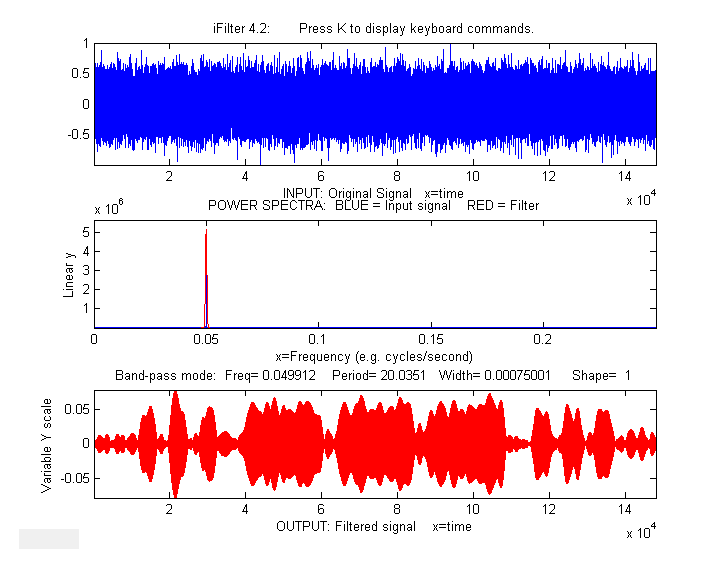frequency (0.05) sine wave that spells out “SOS” in Morse code (dit-dit-dit/dah-dah-dah/dit-dit-dit), adds random white noise so that the SNR is extremely poor (about 0.1 in this example). The white noise has a frequency spectrum that is spread out over the entire range of frequencies; the signal itself is concentrated mostly at a fixed frequency (0.05) but the presence of the Morse Code pulses spreads out its spectrum over a narrow frequency range of about 0.0004. This suggests that a Fourier bandpass filter tuned to the signal frequency might be able to isolate the signal from the noise. As the bandwidth is reduced, the signal-to-noise ratio improves and the signal begins to emerges from the noise until it becomes clear, but if the bandwidth is too narrow, the step response time is too slow to give distinct “dits” and “dahs”. The step response time is inversely proportional to the bandwidth. (Use the ? and " keys to adjust the bandwidth. Press 'P' or the Spacebar to hear the sound). You can actually hear that sine wave component better than you can see it in the waveform plot (upper panel), because the ear works like a spectrum analyzer, with separate nerve endings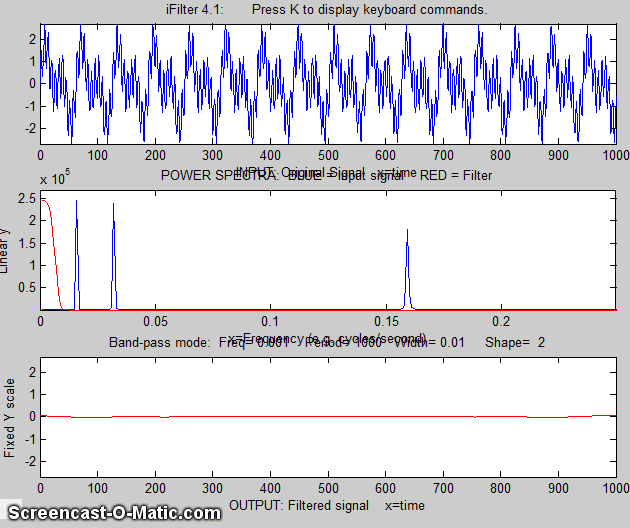assigned to to specific frequencies, whereas the eye analyzes the graph spatially, looking at the overall amplitude and not at individual frequencies.

The script RealTimeFourierFilter.m is a demonstration of a real-time Fourier filter which is discussed in Appendix Y.